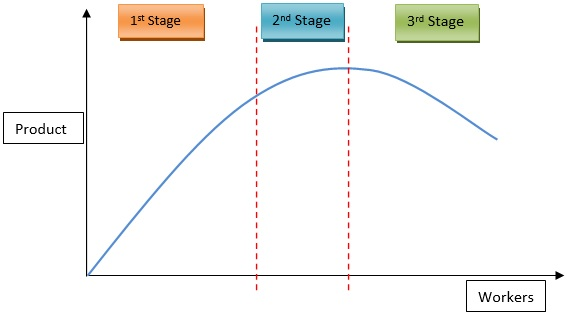# Law of Variable Proportions

Posted in Finance, Accounting and Economics Terms, Total Reads: 313

## Definition: Law of Variable Proportions

Law of Variable proportions deals with the change in output or production function of a firm when one input factor is allowed to vary when all other input factors are kept constant. Law of Variable proportions states that when the value of the variable input factor increases, the proportion of the factor to all other constant input factors also increases and the production function should rise. But what is seen is that first the marginal and then the average product of that factor diminishes. The marginal output obtained by the increase in the variable input factor starts diminishing after certain point. This is known as the Law of Variable proportions.

The Law of Variable proportions follows some assumptions which are

1. Constant level of Technology over the period

2. Variable Factor proportions

3. Factor units are homogenous and hence could be increased by 1 homogenous unit only

4. Valid in the short run because in the long run, the fixed factors become variable and there are other changes that impact production

The concept of Law of Variable proportions is particularly useful in areas of agriculture and manufacturing but is universal across sectors.

The diagram of the production function is shown below:## Law of Variable Proportions Working

1. Under utilization of fixed factors: In the initial stages of production, most of the fixed factors of production are under-utilized. So, more units of the variable factor are needed for the proper or optimal utilization of these factors. And hence, there is increasing returns to additional input.

2. Fixed factors of production: In the short period, some factors are fixed. When the fixed factor is used with the variable factor, then its proportion falls. As production function is a combination of all factors, the marginal return of the variable factor starts declining.

3. Optimum Ratio: There is an optimum ratio of fixed to variable factors that gives maximum utilization and hence maximum marginal production. When the variable factors are increased further, the ratio no longer remains optimum and there is less fixed factors compared to variable factors. This leads to a fall in marginal production.

4. Imperfect Substitutes: After the optimum use of the fixed factors, the increase in variable factors leads to diminishing marginal returns because the fixed factor is not substitutable by other factors. If the fixed factors could be perfectly substituted by other factors, the ratio should remain optimum and the marginal production shall continue to grow. But this does not happen.

## Similarity Between Law of Variable Proportions and Law of Diminishing Returns

Law of Diminishing Returns brings out the same proposition that after a certain point, any additional input actually diminishes the marginal increment in production or output. The average production also falls. This law is highly significant in economics and for firms to identify the optimum point where the marginal return is the highest. It is also called as diminishing marginal returns.

## Example of Law of Variable Proportions

Let us consider a situation when there are two input factors- Land and Labour. We assume that land is the fixed factor whereas labor is the variable input factor. The law states that there are 3 major stages that the production function passes through when we keep on increasing the labor input by 1 unit, based on marginal production (MP) and average production (AP). In stage 1, MP>AP, In stage 2, MP<AP and in stage 3, MP<0. Increasing labor input by 1 unit in fact reduces the output.

 UNITS OF LAND UNITS OF LABOUR TOTAL PRODUCTION AVERAGE PRODUCTION MARGINAL PRODUCTION 10 Acres 0 1 2 3 4 5 6 7 8 20 50 90 120 140 150 150 140 20 25 30 30 28 25 21.3 17.5 20 30 40 30 20 10 0 -10 MP>AP MP=AP MP

Hence, this concludes the definition of Law of Variable Proportions along with its overview.

Browse the definition and meaning of more terms similar to Law of Variable Proportions. The Management Dictionary covers over 7000 business concepts from 6 categories.

Search & Explore : Management Dictionary

Similar Definitions from same Category: